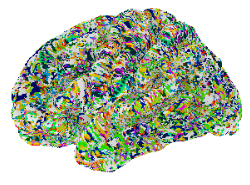Dear All,

I am having some difficulty in using the Gordon parcellation (https://sites.wustl.edu/petersenschlaggarlab/parcels-19cwpgu/) for surface based connectivity (specifically the 164K surface version)

If I view this parcellation using connectome workbench it looks as one would expect:

However if I view it using nilearn:

``````from nilearn import plotting, surface, datasets
fsaverage = datasets.fetch_surf_fsaverage(mesh='fsaverage')
plotting.plot_surf_roi(fsaverage['pial_left'], roi_map=parcellation,
hemi='left', view='lateral',
bg_map=fsaverage['sulc_left'], bg_on_data=True,
darkness=.5)
``````

The rois are broken up and shuffled across the cortex,This is reflected in the fact that when I try and build a connectivity matrix the expected structure is not seen.

``````def surface_conn(sub_id, denoised_path):
atlas_l_path = f'/home/k1201869/glu_rs/data/atlas_data/gordon/notworking'
atlas_r_path = f'/home/k1201869/glu_rs/data/atlas_data/gordon/notworking'
surf_image_l_path = f'{denoised_path}/sub-{sub_id}/regress/lh.sub-{sub_id}_residualised.mgz'
surf_image_r_path = f'{denoised_path}/sub-{sub_id}/regress/rh.sub-{sub_id}_residualised.mgz'

l_roi_timecourses = []
# We don't include the first roi as this is background
for i in np.unique(atlas_image_l)[1:]:
roi_idxs = np.where(atlas_image_l==i)
l_roi_timecourses.append(np.mean(surf_image_l[roi_idxs], axis=0))

r_roi_timecourses = []
for i in np.unique(atlas_image_r)[1:]:
roi_idxs = np.where(atlas_image_r==i)
r_roi_timecourses.append(np.mean(surf_image_r[roi_idxs], axis=0))

bilat_timecourses = np.array(l_roi_timecourses + r_roi_timecourses)
connectivity_matrix = np.corrcoef(bilat_timecourses)

return connectivity_matrix
``````

So it seems as though when loading the parcellation with nilearn the vertices are getting shuffled, I wonder if anyone has any advice about how to fix this?

Thanks!

Rob

Edit: I think I just need to convert from HCP to FS space following the steps here: https://figshare.com/articles/HCP-MMP1_0_projected_on_fsaverage/3498446

I’d agree with the path you suggested in your edit ! To add a bit more context: nilearn currently only works with giftis in FS space, so it wouldn’t recognize the same information in a `dlabel.nii` that ConnectomeWB will.

I think the steps outlined in the figshare link you shared are generally right, although I don’t think you’ll want to do the final step (converting to `.annot`), and instead just pass the gifti files !

Let us know how it goes !

My understanding is that the connectome WB mesh is not the fsaverage one, so you would need to resample the ROI definifiotn on fsaverage7 mesh. Is this what you mean @emdupre ?

Yes, exactly ! The figshare link contains instructions for projecting the HCP-MMP1.0 parcellation from fsLR (used across HCP) onto fsaverage (the high resolution, 164k vertices mesh).

As a follow-up: reviewing the code, I think you’re right @rob that `plot_surf_roi` should take the `.annot` file as an `roi_map` argument. So I’ll be curious to know if the updated files work for you !

Thanks for the input!

A few less steps are required than in the figshare. Instructions below for right hemisphere:

1. Download `R.sphere.32k_fs_LR.surf.gii` from

and `R-to-fs_LR_fsaverage.R_LR.spherical_std.164k_fs_R.surf.gii` from

Run:

`wb_command -label-resample Parcels_R.func.gii R.sphere.32k_fs_LR.surf.gii fs_R-to-fs_LR_fsaverage.R_LR.spherical_std.164k_fs_R.surf.gii BARYCENTRIC right.fsaverage164.label.gii`

2 Likes

Hello,

I’m a bit confused about the wb_command commands to resample an hcp cifti surface data (not labels) to fsaverage, and vice-versa. I’m trying to follow the basic commands done here: https://github.com/netneurolab/markello_spatialnulls/blob/master/scripts/empirical/fetch_hcp_myelin.py

After

``````wb_command -cifti-separate rfMRI_REST1_LR_Atlas_stats.dscalar.nii COLUMN -metric CORTEX_LEFT cor_left.func.gii -metric CORTEX_RIGHT cor_right.func.gii
``````

I believe I need to apply the following command:

``````wb_command -metric-resample cor_right.func.gii ??
``````

But I’m confused which files I need to use as the current sphere and new sphere options?

Tom

Hi @tomvanasse !

The HCP provides instructions on how to map between fsLR and fsaverage space in their wiki here.

The document linked in that answer (document link) provides step-by-step instructions on which files should go where in the `wb_command -metric-resample` command, as well as a link to where you can download the relevant template files.

Hope that helps !
Ross

1 Like

MANAGED BY INCF